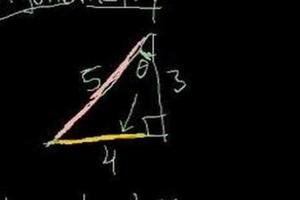# Math## MATH

• CCSS ALGEBRA I
• CCSS GEOMETRY
• CCSS ALGEBRA 2 + PRE-CALCULUS COMPRESSION
• PROBABILITY & STATISTICS
• AP CALCULUS AB
• AP STATISTICS

CCSS ALGEBRA I

DESCRIPTION

This course was specifically developed to address the Common Core State Standards for Mathematics, especially the Standards of Mathematical Practice. The fundamental goal is for all students to make sense of the mathematics they are learning, and for this reason all units are designed around four challenging tasks connected by lesson series of a varying number of days. The tasks require that students find patterns, make and test conjectures, use multiple representations and approaches, make generalizations, and verify that solutions are reasonable in a given context. The lesson series are designed to balance conceptual understanding, modeling, and procedural fluency.

CCSS GEOMETRY

PREREQUISITE Algebra I

DESCRIPTION

(Required for all 10th graders, unless the course was completed by a summer program pre-approved by RA SOTA counseling department)

This is the second mathematics course for high school students aligned to the Common Core State Standards for Mathematics. The course begins with transformations in the plane, developing the concept of congruence through rigid motions and the concept of similarity through dilations. Relationships established through transformational geometry are used to prove geometric theorems, including theorems involving circles. Similarity leads to a study of right triangles and trigonometric ratios. A focus on expressing geometric properties with equations leads to using coordinates to prove theorems algebraically. The course also examines relationships between 2- and 3-dimensional objects, including volume formulas, and modeling with geometry.

Graduation Requirement: All SFUSD students are required to complete:

• CCSS Algebra I
• CCSS Geometry
• CCSS Algebra 2 or CCSS Algebra 2 + Precalculus compression

Students that have completed three years of the required math can be eligible for a math elective but priority will be given to seniors.

PREREQUISITE  Algebra I and Geometry

CCSS ALGEBRA 2 (Required unless enrolled in Algebra 2 + Precalculus Compression)

This is the third course for high school students aligned to the Common Core State Standards.  The course continues the previous work on functions that include: building a function that model a relationship between two quantities and building new functions from existing functions (linear and non-linear); constructing and comparing linear and exponential models; understanding trigonometric functions (sine and cosine), unit circle, modeling periodic phenomena, and proving and applying Pythagorean Identity; using polynomial identities, rewriting rational functions; understanding random processes, statistical experiments, and making inferences and justifying conclusions; understanding conditional probability, rules of probability, compound events, expected value, and using probability to evaluate outcomes of decisions.

CCSS ALGEBRA 2 + PRECALCULUS COMPRESSION (Optional but fulfills Algebra 2 graduation requirement. Strong algebra skills recommended)

This is the third course for high school students aligned to the Common Core State Standards. The course has a strong focus on expanding students' understanding of functions and generalizing function properties to transform a variety of functions, including:  constructing and comparing linear and exponential models; transforming and modeling situations with a variety of function families (including quadratic, square root, absolute value); understanding the unit circle and trigonometric functions (sine, cosine, tangent, and the reciprocal functions), modeling periodic phenomena; working with polynomial and rational expressions and functions. In statistics, topics include understanding the properties of the normal curve, random processes, statistical experiments, and making inferences and justifying conclusions. This course covers additional topics beyond Algebra 2 to prepare students for AP Calculus, which include the following: trigonometric identities, composition of functions, piecewise functions, logarithmic functions, and inverse functions.

Students that have completed all SFUSD Math requirements (Algebra 1, Algebra 2 and Geometry) can take one of the following Math electives on campus in their senior year (Please note: AP CALCULUS requires a completion of the Compression Course with a grade of C or higher)

PROBABILITY & STATISTICS (Math elective)

Probability & Statistics introduce students to the major concepts and tools for collecting, analyzing, and drawing conclusions from data. This course emphasizes conceptual understanding of data analysis, with a focus on the statistical thinking that underlies data gathering and interpretation. Students learn to describe and model data distributions, describe relationships between variables, and design and apply different methods for gathering data. Students also study probability and probability models.

AP STATISTICS (Math elective)

The AP Statistics course is equivalent to a one-semester, introductory, non-calculus-based college course in statistics. The course introduces students to the major concepts and tools for collecting, analyzing, and drawing conclusions from data. There are four themes in the AP Statistics course: exploring data, sampling and experimentation, anticipating patterns, and statistical inference. Students use technology, investigations, problem solving, and writing as they build conceptual understanding. Appropriate for students with college and career goals in many fields, including humanities, sciences, and art. Students with a goal related to engineering are encouraged to take AP Calculus.

AP CALCULUS AB (Math elective - Must have completed Compression course to take this course)

Building enduring mathematical understanding requires understanding the why and how of mathematics in addition to mastering the necessary procedures and skills. To foster this deeper level of learning, AP Calculus AB is designed to develop mathematical knowledge conceptually, guiding you to connect topics and representations throughout the course and to apply strategies and techniques to accurately solve diverse types of problems.